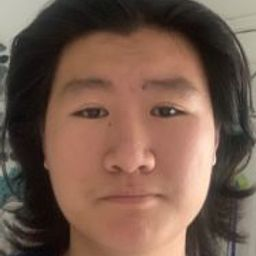🤔 Find out what you don't know with free Quizzes 🤔Start Quiz Now!# Evaluate $\int_{C}(x+y) d s,$ where $C$ is the straight-line segment $x=t, y=(1-t), z=0,$ from (0,1,0) to (1,0,0).

## $\sqrt{2}$

Integrals

Vectors

Vector Functions

### Discussion

You must be signed in to discuss.
##### Top Calculus 3 Educators##### Heather Z.

Oregon State University##### Kristen K.

University of Michigan - Ann Arbor##### Michael J.

Idaho State University

Lectures

Join Bootcamp

### Video Transcript

Okay, folks. So in this video, we're gonna take a look at this line intro. So we have the line integral along the curve C of picks. Plus why, yes. And what we're given is were given, um X and Y each as a function of t X as a function of t is just tea. And why, as a function of t gives you one minus t. So the way we're gonna do this Integral is by is by rewriting the DS as a function of t. So let me remind you what Ds is, DS. If you have a three dimensional space, DS is just a line on integral Excuse me on infinite testable lying segment. And as you know, we'll never you have a lying second. You can write it as a function of X, y and Z, but in this case, we don't have a Z component, so we don't have to worry about. See, we're just gonna ride the I squared as the X squared plus d y squared. Normally there would be a D Z squared as well, but in this case, we don't have it, so we don't worry about it. So now What we're gonna do is we're gonna take care of the square roots. I mean, take care of the square by square rooting both sides we have DS equals the square root of D X squared plus d y squared. And then what we're gonna do is we're gonna do a little bit of trick here. We're gonna divide by DT inside the square root and then multiply it back. So we have this thing is really this thing right here is really just the X over d t squared plus the y over DT squared and then bracket multiplied by d t squared. OK, um and now, right now, all of this is just algebra. Now I can pull the DT out of the square root and then I have x dot squared plus y dot squared. I hope you know what next? On why DOT is just notation x dot means the time derivative of X with respect ity. Okay, When you pull the key squared out of the out of the square root, you get his DT right get just DT and then you have DS on the other side. So another worst. We have successfully re parameter rised the variable s as a function of t. So now what we're gonna do is we're gonna evaluate x dot Which is just, you know, dx DT. And you know what X is X S t excess liquidity. So DX DT is just one okay. And what about why dot why dies A of is the time derivative of why, um So you get d u by d t of one minus t. Which is gonna give you negative one so we can pluck these two back in and we'll get the s equals ex top square to just one. Why got squares also one multiplied by DT. So that's just rude to DT. And then now gonna plug this whole thing back into the Inter girls. We have this integral see and then extra Plus why? Which I'm going to write as a function of t so t plus why? Why is one minus t Okay, so now we do the s what's dsps as a route to DT. Okay, now, that's very nice, because you see that t and T here are gonna cancel. Now you have one times Route two, which is just rude to which I'm gonna pull out of Of the Integral Now I have didn't grow of DT, and that's they're easy to do. This is just route to of tea evaluated at the two. Ah, endpoints. What's the first endpoint was the second point. Well, the first endpoint, Um which is, which is, um which is when X y z equals 010 And the other end point is when x y z equals 100 But X, Y and Z or both are all functions. Sometimes we're gonna rewrite this these limits as functions of time when X is one that means that means two years one Okay, when x zero when x zero. That means t is zero, right? Let me just do a quick check here. The 1st 1.10 and when? Why is one that means one minus t is one so t zero? That's good. So we have we have are two endpoints are to limits that were evaluating this inter grow in. Now we have. All we gotta do is we gotta do the usual, you know, plugging in and plug and chug and all that kind of stuff. We have t when t is one. T is also one minus 20020 So we have room to. So the answer to this integral this line into grow is route two. All right. I think that's it for this video. Thank you. And I'll see you in the next video byUniversity of California, Berkeley

#### Topics

Integrals

Vectors

Vector Functions

##### Top Calculus 3 Educators##### Heather Z.

Oregon State University##### Kristen K.

University of Michigan - Ann Arbor##### Michael J.

Idaho State University

Lectures

Join Bootcamp